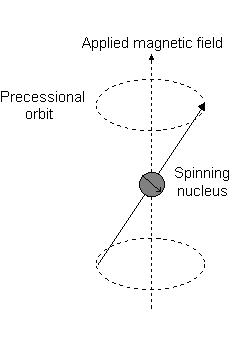# What Is Z component of orbital angular momentum? How can we find the Z component? What is its importance? What does it resemble?

Dec 21, 2016

You seem to be referring to ${m}_{l}$, which is the observed value that corresponds to the $z$-component of the total orbital angular momentum ${L}_{z}$.

Practically speaking, for general chemistry, you can simply use the value of $l$ as the range of ${m}_{l}$, and express ${m}_{l}$ as:

$\boldsymbol{{m}_{l} = \left\{- l , - l + 1 , . . . , 0 , . . . , l - 1 , l\right\}}$

For instance, if $l = 2$ (as for a $d$ orbital), then:

${m}_{l} = \left\{- 2 , - 1 , 0 , + 1 , + 2\right\}$

That means five $d$ orbitals exist for a given principal quantum number $n$:RELATION TO THE Z-COMPONENT OF THE TOTAL ORBITAL ANGULAR MOMENTUM

Recall that the Schrodinger equation is typically written as $\hat{H} \psi = E \psi$ (where $E$ is the energy, $\hat{H}$ is the Hamiltonian operator, and $\psi$ is the wave function).

Well, it turns out that $\psi$, the wave function describing the state of a quantum mechanical system, can be separated into a radial and an angular component, ${R}_{n l} \left(r\right)$ and ${Y}_{l}^{{m}_{l}} \left(\theta , \phi\right)$:

${\psi}_{n l {m}_{l}} \left(r , \theta , \phi\right) = {R}_{n l} \left(r\right) {Y}_{l}^{{m}_{l}} \left(\theta , \phi\right)$

where $n$, $l$, and ${m}_{l}$ are the principal, angular momentum, and magnetic quantum numbers, respectively.

Traditionally, ${m}_{l}$ is defined to be the $z$ component of the angular momentum $l$, and it is the eigenvalue (the quantity we expect to see over and over again), in units of ℏ, of the wave function, $\psi$.

This eigenvalue corresponds to the operator for ${L}_{z}$, and ${L}_{z}$ is the $\boldsymbol{z}$ component of the total orbital angular momentum.

What we just said can be expressed as:

$\stackrel{\text{Operator")overbrace(hatL_z)" "stackrel("Angular")stackrel(" Component")stackrel("of Wave Function")overbrace(Y_(l)^(m_l)(theta,phi)) = stackrel("Eigenvalue")overbrace(m_lℏ)" "stackrel("Angular")stackrel(" Component")stackrel("of Wave Function}}{\overbrace{{Y}_{l}^{{m}_{l}} \left(\theta , \phi\right)}}$

If ${L}_{z}$ is what you mean, then the significance of it is that it is the phenomenon we can observe that corresponds to the magnetic quantum number ${m}_{l}$.

PHYSICS PERSPECTIVE

Visually, in the presence of a magnetic field in the $z$ direction, a nuclear rotation (exhibiting a total orbital angular momentum) occurs along the $z$ axis, called a "Larmor precession".

This is the event described by ${L}_{z}$.

For instance, when $l = 1$, as for a $p$ orbital, ${m}_{l} = \left\{- 1 , 0 , + 1\right\}$. The "Larmor precession" that occurs looks like the following for a $2 {p}_{z}$ orbital:And each ${m}_{l}$ corresponds to the distance from the $z$ axis in units of ℏ:For instance:

• An ${m}_{l}$ of $1$ corresponds to the upper half of the $2 {p}_{z}$ orbital.
• An ${m}_{l}$ of $0$ is the dot at the origin.
• An ${m}_{l}$ of $- 1$ corresponds to the lower half.

CHEMISTRY PERSPECTIVE

From a practical point of view, what we really care about is how to use ${m}_{l}$. Each ${m}_{l}$ corresponds to a unique orbital in a particular subshell. So:

• The number of ${m}_{l}$ values tells you the number of orbitals in a subshell.
• The range of ${m}_{l}$ is based on the chosen $l$.

For example, since $l = 2$ is for a $d$ subshell, then:

${m}_{l} = \left\{- 2 , - 1 , 0 , + 1 , + 2\right\}$

That means five $d$ orbitals exist for a given principal quantum number $n$: